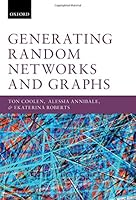# Generating Random Networks and Graphs## Book Description

Generating random efficiently and accurately is an important challenge for practical applications, and an interesting question for theoretical study. This book presents and discusses common methods of generating random graphs. It begins with approaches such as Exponential Random Models, where the targeted probability of each appearing in the ensemble is specified. This section also includes degree-preserving randomisation algorithms, where the aim is to generate networks with the correct number of links at each , and care must be taken to avoid introducing a bias. Separately, it looks at growth style algorithms (e.g. preferential attachment) which aim to model a real process and then to analyse the resulting ensemble of graphs. It also covers how to generate special types of graphs including modular graphs, graphs with community structure and temporal graphs.

The book is aimed at the graduate student or advanced undergraduate. It includes many worked examples and open questions making it suitable for use in teaching. Explicit pseudocode algorithms are included throughout the book to make the ideas straightforward to apply.

With larger and larger datasets, it is crucial to have practical and well-understood tools. Being able to test a hypothesis against a properly specified control case is at the heart of the 'scientific method'. Hence, knowledge on how to generate controlled and unbiased random graph ensembles is vital for anybody wishing to apply network science in their research.

Part I The basics
Chapter 1 Introduction
Chapter 2 Definitions And Concepts
Chapter 3 Random Graph Ensembles

Part II Random graph ensembles
Chapter 4 Soft Constraints: Exponential Random Graph Models
Chapter 5 Ensembles With Hard Constraints

Part III Generating graphs from graph ensembles
Chapter 6 Markov Chain Monte Carlo Sampling Of Graphs
Chapter 7 Graphs With Hard Constraints: Further Applications And Extensions

Part IV Graphs defined by algorithms
Chapter 8 Network Growth Algorithms
Chapter 9 Specific Constructions

Part V Further topics
Chapter 10 Graphs On Structured Spaces

Appendix A The delta
Appendix B Clustering and correlations in Erdös–Rényi graphs
Appendix C Solution of the two-star exponential random graph model (ERGM) in the sparse regime
Appendix D Steepest descent integration
Appendix E Number of sparse graphs with prescribed average degree and degree variance
Appendix F Evolution of graph mobilities for edge-swap
Appendix G Number of graphs with prescribed degree sequence
Appendix H Degree correlations in ensembles with constrained degrees
Appendix I Evolution of triangle and square counters due to ordered edge swaps
Appendix J Algorithms